Next: Complex Zernike moments Up: Orthogonal moments Previous: Orthogonal moments

## Legendre moments

The Legendre moments  of order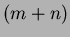are defined as: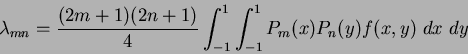(45)

where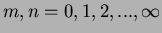,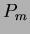and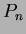are the Legendre polynomials and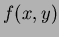is the continuous image function. The Legendre polynomials are a complete orthogonal basis set defined over the interval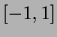. For orthogonality to exist in the moments, the image functionis defined over the same interval as the basis set, where the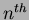order Legendre polynomial is defined as: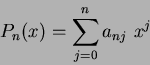(46)

and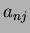are the Legendre coefficients given by: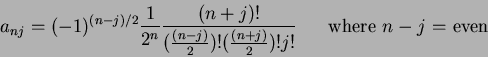(47)

So, for a discrete image with current pixel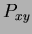, Equation 1.45 becomes: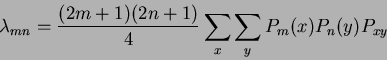(48)

and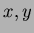are defined over the interval.

Jamie Shutler 2002-08-15